# 迈出Pycharm第一步

## 一、创建并运行你的第一个Python工程

### 3.创建一个纯Python工程

Python提供了一系列的工程模版，用于创建不同类型的应用。比如Django、Google AppEngine等等。通过这些模版，Pycharm会自动创建相应的目录结构和特殊文件以及运行配置。

• 点击location后面的按钮，选择你希望的工程位置目录。在这个界面里，你可以对文件系统进行浏览和操作，比如创建新文件夹等等。
• 点开`Project Interpreter: New Virtualenv Environment`，选择一个用于创建虚拟环境的工具，通常使用Virtualenv。指定虚拟环境的生成位置以及被虚拟的Python解释器。两个可选项，第一个是继承全局库文件，通常不选，第二个是对所有工程有效，通常也不选。
• 也可以使用下面的Existing interpreter，选择一个已经存在的解释器。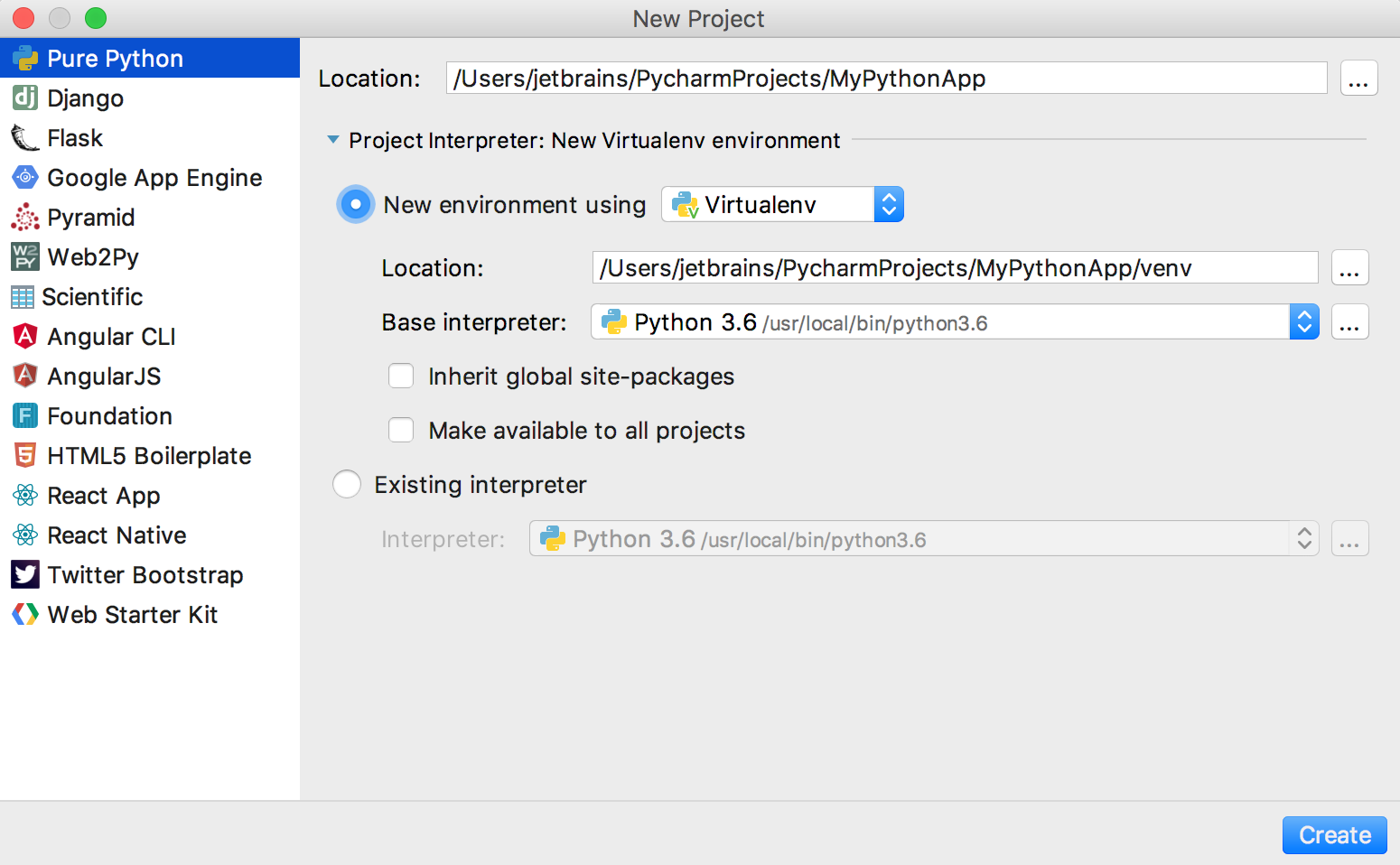### 4.创建Python文件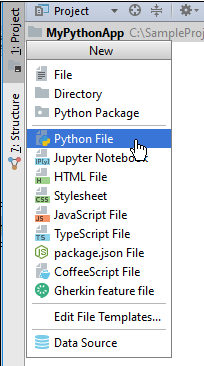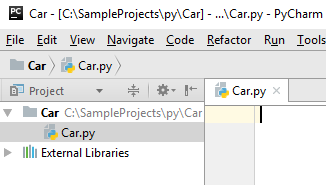### 5.编辑源代码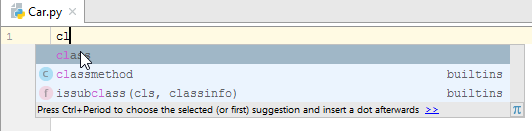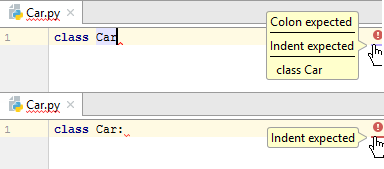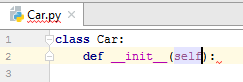```class Car:
def __init__(self):
self.speed = 0
self.odometer = 0
self.time = 0

def say_state(self):
print("I'm going {} kph!".format(self.speed))

def accelerate(self):
self.speed += 5

def brake(self):
self.speed -= 5

def step(self):
self.odometer += self.speed
self.time += 1

def average_speed(self):
return self.odometer / self.time

if __name__ == '__main__':
my_car = Car()
print("I'm a car!")
while True:
action = input("What should I do? [A]ccelerate, [B]rake, "
"show [O]dometer, or show average [S]peed?").upper()
if action not in "ABOS" or len(action) != 1:
print("I don't know how to do that")
continue
if action == 'A':
my_car.accelerate()
elif action == 'B':
my_car.brake()
elif action == 'O':
print("The car has driven {} kilometers".format(my_car.odometer))
elif action == 'S':
print("The car's average speed was {} kph".format(my_car.average_speed()))
my_car.step()
my_car.say_state()
```

### 6.运行程序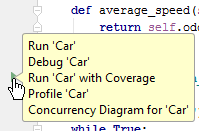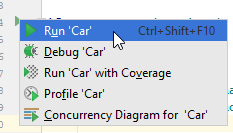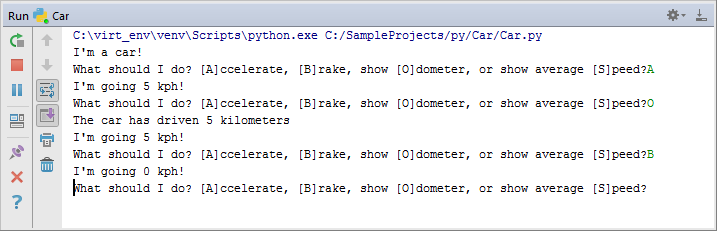### 7.配置运行/调试参数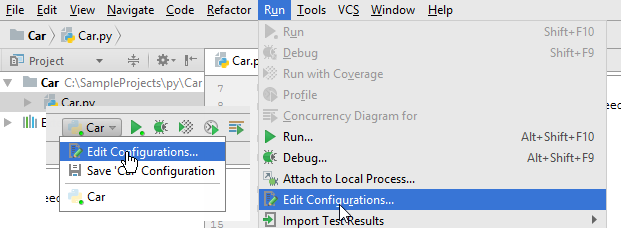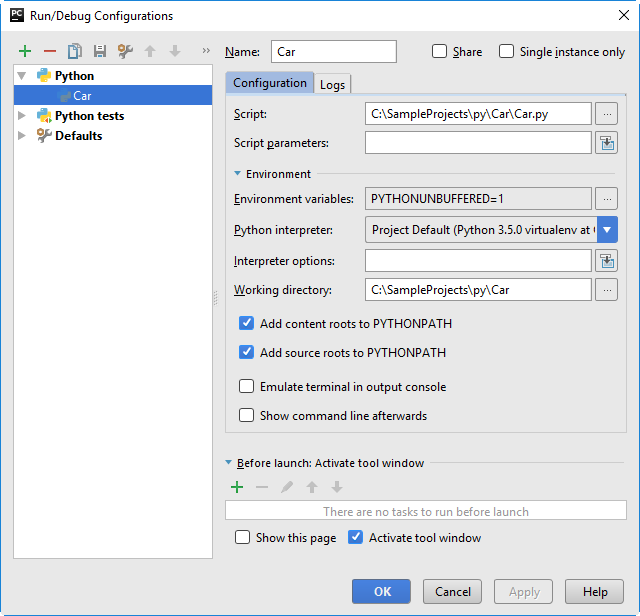## 二、调试Python应用

### 1. 查找问题的出处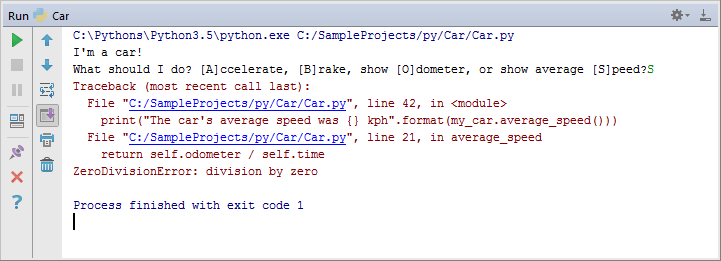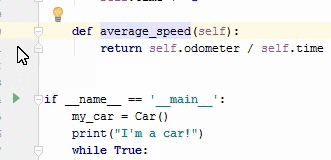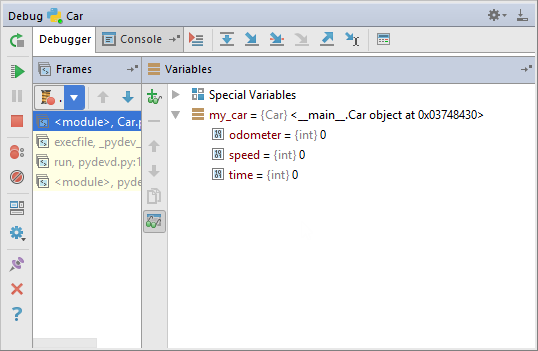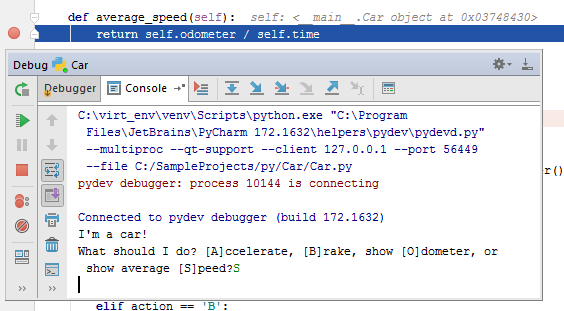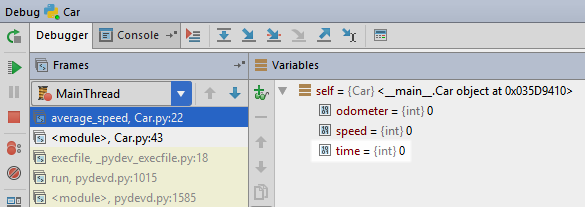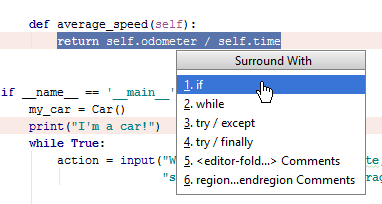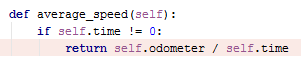### 2.调试的细节

• 步进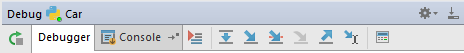• 监控watching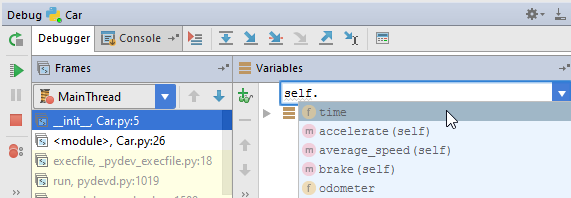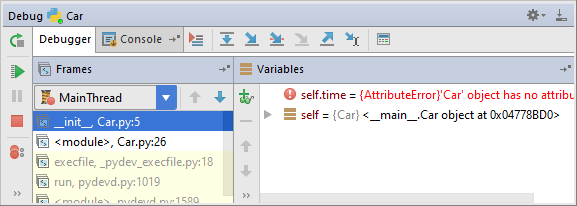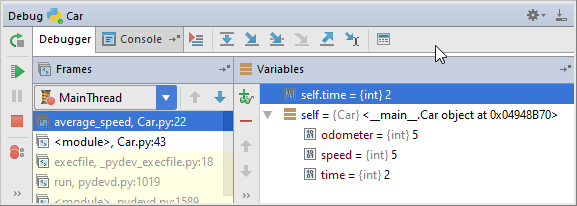• 行内提示信息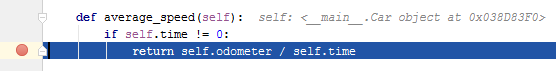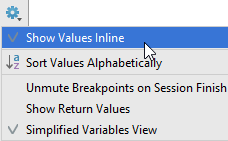• 执行语句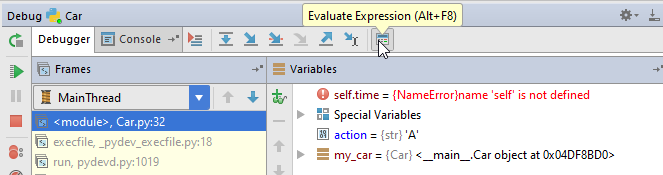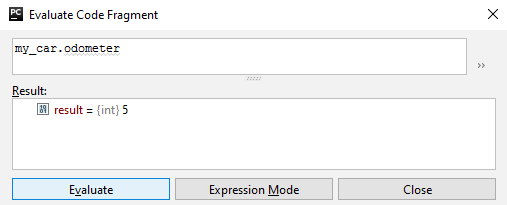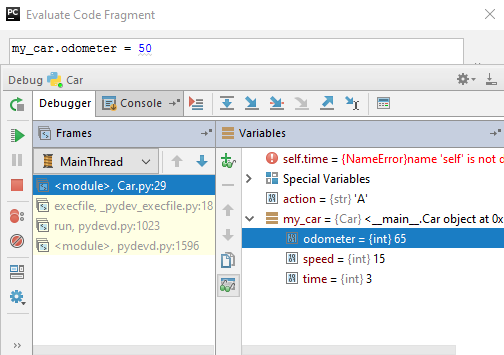## 三、测试Python应用程序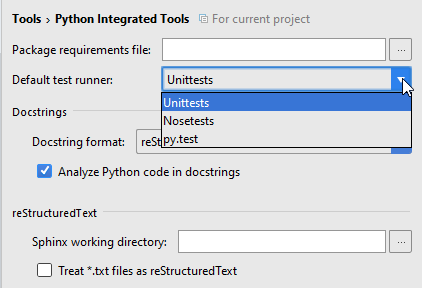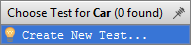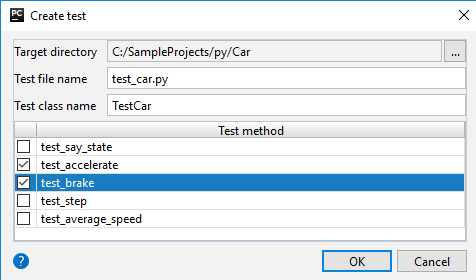Pycharm会自动生成一个新的名为test_car.py的测试脚本，如下图 所示：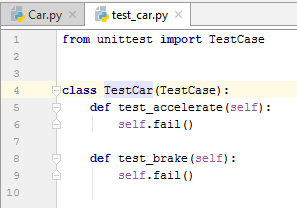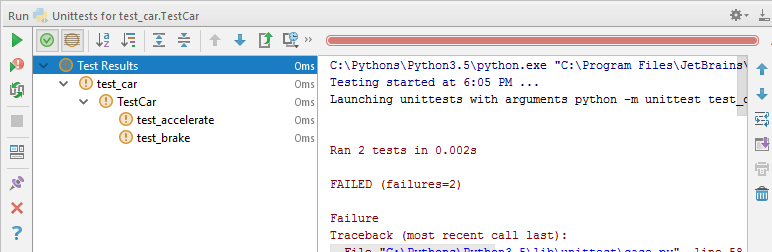```import unittest

from Car import Car

class TestCar(unittest.TestCase):
def setUp(self):
self.car = Car()

class TestInit(TestCar):
def test_initial_speed(self):
self.assertEqual(self.car.speed, 0)

def test_initial_odometer(self):
self.assertEqual(self.car.odometer, 0)

def test_initial_time(self):
self.assertEqual(self.car.time, 0)

class TestAccelerate(TestCar):
def test_accelerate_from_zero(self):
self.car.accelerate()
self.assertEqual(self.car.speed, 5)

def test_multiple_accelerates(self):
for _ in range(3):
self.car.accelerate()
self.assertEqual(self.car.speed, 15)

class TestBrake(TestCar):
def test_brake_once(self):
self.car.accelerate()
self.car.brake()
self.assertEqual(self.car.speed, 0)

def test_multiple_brakes(self):
for _ in range(5):
self.car.accelerate()
for _ in range(3):
self.car.brake()
self.assertEqual(self.car.speed, 10)

def test_should_not_allow_negative_speed(self):
self.car.brake()
self.assertEqual(self.car.speed, 0)

def test_multiple_brakes_at_zero(self):
for _ in range(3):
self.car.brake()
self.assertEqual(self.car.speed, 0)
```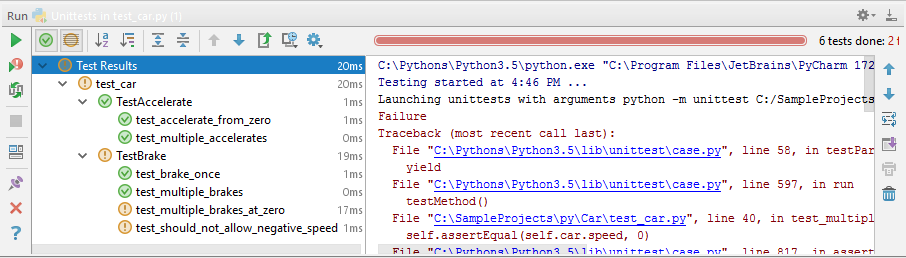Pycharm可以自动运行测试代码，你只需要做两件事：

1. 点击运行工具栏中的Toggle（绿色三角箭头下的第二个，两个灰框，有循环箭头的图标），激活自动测试功能
2. 点击工具栏中的蓝色齿轮图标，选择`Set Autotest Delay`，然后选择延迟时间。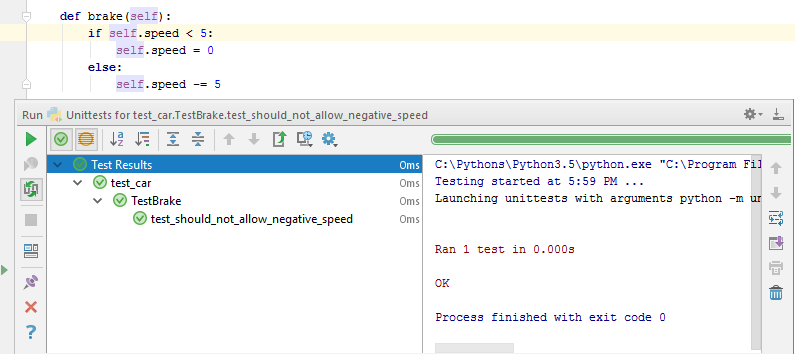## 四、创建并运行Django工程

### 1.创建新工程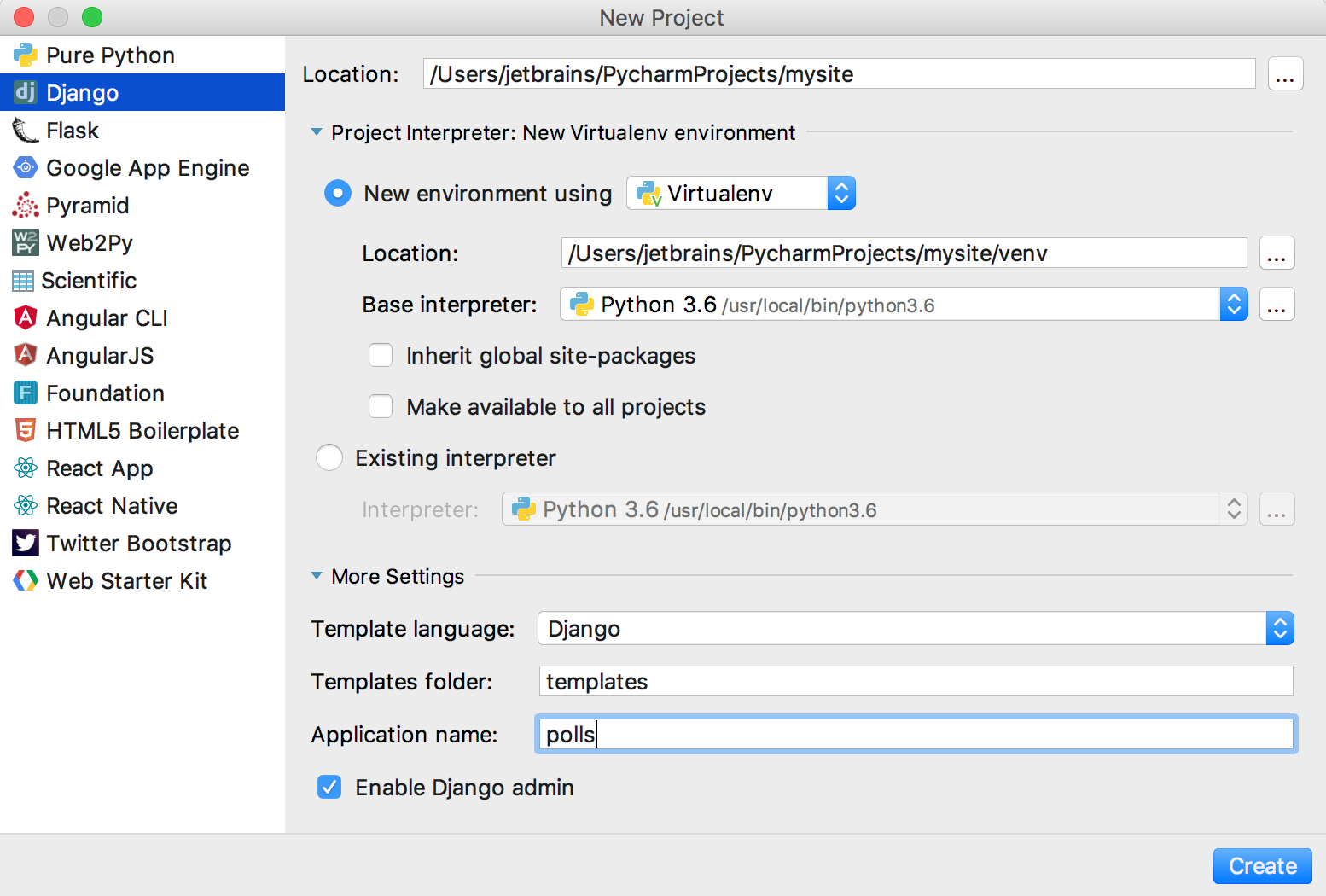（详细介绍下所有的设定参数）

### 2.浏览项目结构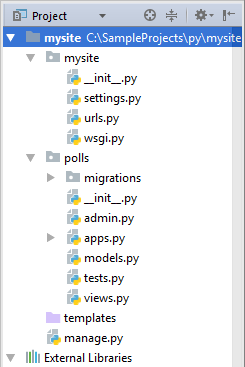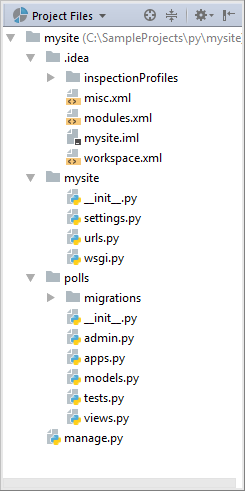（介绍一下所有文件和目录的作用）

`Tools | Run manage.py task`菜单，可以键入Django manage.py所支持的各种命令。

### 3.配置数据库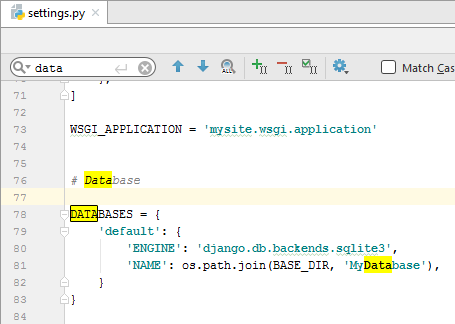### 4.运行Django服务器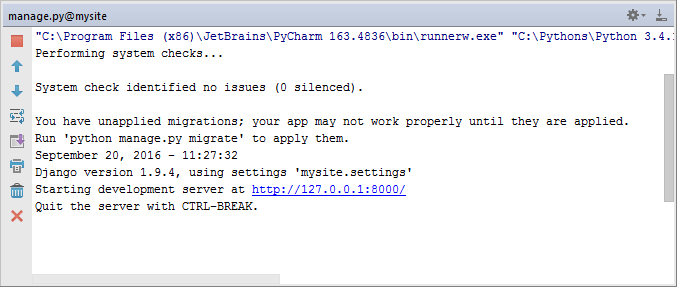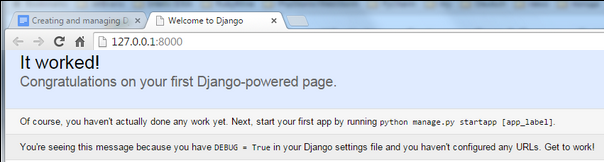### 5.创建模型

```from django.db import models

# the following lines added:
import datetime
from django.utils import timezone

class Question(models.Model):
question_text = models.CharField(max_length=200)
pub_date = models.DateTimeField('date published')

def __str__(self):
return self.question_text

def was_published_recently(self):
now = timezone.now()
return now - datetime.timedelta(days=1) <= self.pub_date <= now

was_published_recently.admin_order_field = 'pub_date'
was_published_recently.boolean = True
was_published_recently.short_description = 'Published recently?'

class Choice(models.Model):
question = models.ForeignKey(Question, on_delete=models.DO_NOTHING,)
choice_text = models.CharField(max_length=200)
votes = models.IntegerField(default=0)

def __str__(self):
return self.choice_text
```

### 6.生成数据表

`manage.py`控制台输入`makemigrations polls`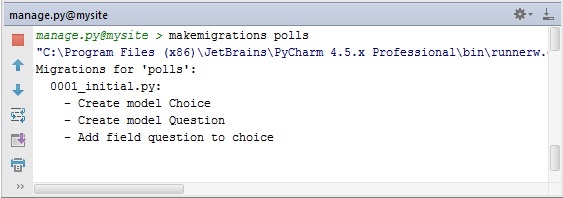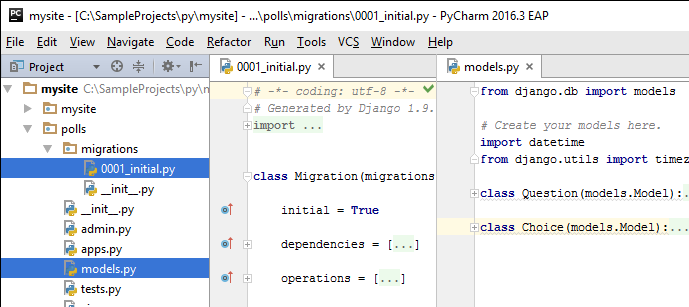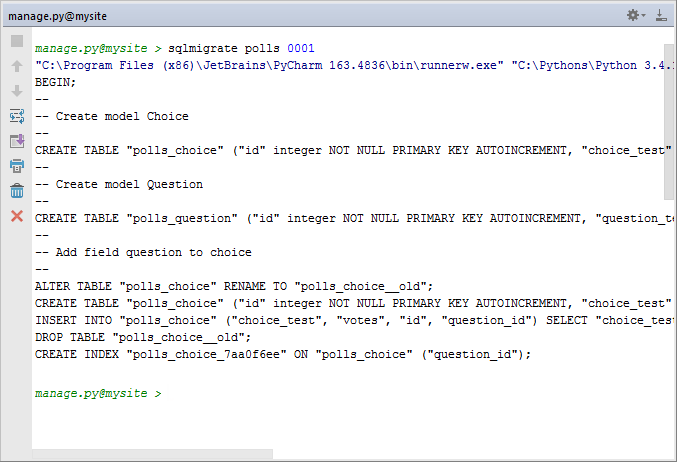### 7.启用admin后台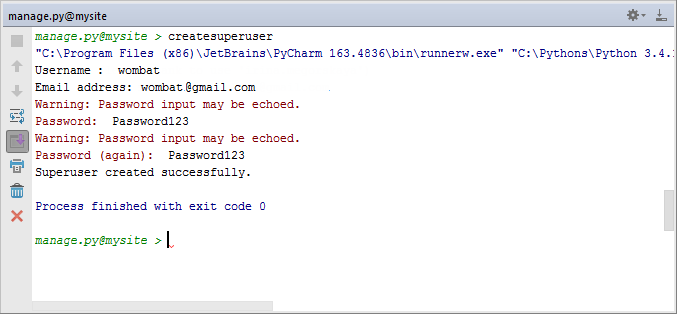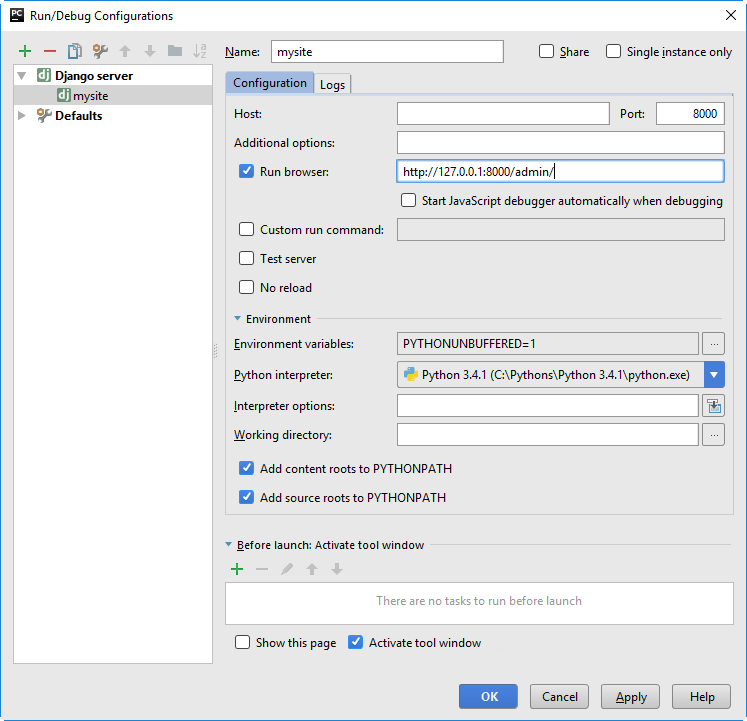（讲解配置参数）

```from django.contrib import admin
from .models import Question #this line added
admin.site.register(Question)#this line added
```
```from django.contrib import admin
from .models import Choice, Question

class ChoiceInline(admin.TabularInline):
model = Choice
extra = 3

class QuestionAdmin(admin.ModelAdmin):
fieldsets = [
(None,               {'fields': ['question_text']}),
('Date information', {'fields': ['pub_date'], 'classes': ['collapse']}),
]
inlines = [ChoiceInline]

admin.site.register(Question, QuestionAdmin)
```

(注册admin，修改admin显示)

### 8.编写视图

views.py

```from django.http import HttpResponse

def index(request):
return HttpResponse("Hello, world. You're at the polls index.")
```

polls/urls.py

```from django.conf.urls import url
from . import views
urlpatterns = [
url(r'^\$', views.index, name='index'),
]
```

urls.py

```from django.conf.urls import include, url
from django.contrib import admin
urlpatterns = [
url(r'^polls/', include('polls.urls')), #this line added
url(r'^admin/', admin.site.urls),
]
``````def detail(request, question_id):
return HttpResponse("You're looking at question %s." % question_id)

def results(request, question_id):
response = "You're looking at the results of question %s."
return HttpResponse(response % question_id)

def vote(request, question_id):
return HttpResponse("You're voting on question %s." % question_id)
```

```from django.conf.urls import url
from . import views

urlpatterns = [
url(r'^\$', views.index, name='index'),
# ex: /polls/5/
url(r'^(?P<question_id>[0-9]+)/\$', views.detail, name='detail'),
# ex: /polls/5/results/
url(r'^(?P<question_id>[0-9]+)/results/\$', views.results, name='results'),
# ex: /polls/5/vote/
url(r'^(?P<question_id>[0-9]+)/vote/\$', views.vote, name='vote'),
]
```### 评论总数： 3### 最新博客:

Django之contenttypes框架
Django REST framework 核心组件： 序列化器
HTTPie--命令行下的HTTP工具

### 推荐阅读:

Django2.1新手图文入门教程 42637
Django1.10新手图文入门教程 23219